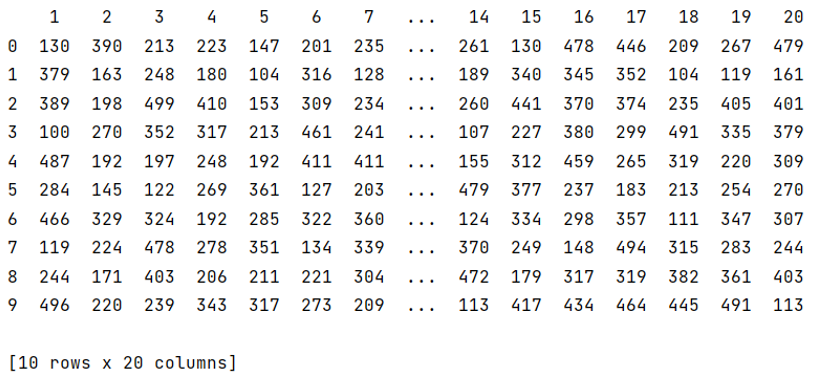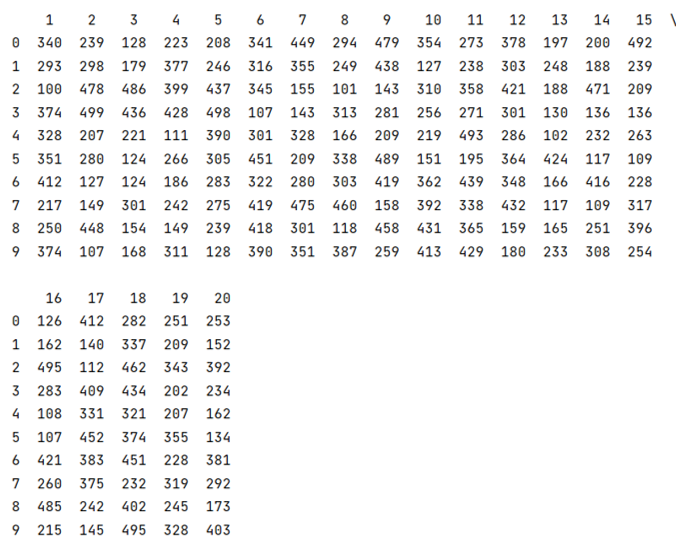# How to show all columns' names on a large Pandas DataFrame?

Given a Pandas DataFrame, we have to show all columns' names. By Pranit Sharma Last updated : September 21, 2023

In the real world, data is huge so is the dataset. While importing a dataset and converting it into DataFrame, if the number of columns is large, the default printing method does not print the entire columns. It compresses the rows and columns. In this article, we are going to learn how to expand the output display to see all the columns of DataFrame.

## Problem statement

Given a Pandas DataFrame, we have to show all columns' names.

## Showing all columns' names on a large Pandas DataFrame

We can achieve this task with the help of pandas.set_option() method. The set_options() method allows us to set different properties according to our requirements. The display.max_columns defines the total number of columns to be printed. If None is passed as an argument, all columns would be printed.

Note

To work with pandas, we need to import pandas package first, below is the syntax:

```import pandas as pd
```

Let us understand with the help of an example.

## Python program to show all columns' names on a large Pandas DataFrame

Here, we have defined 20 columns but not all the columns are printed, to overcome this problem, we will use set_options('display.max_columns') method.

```#  Importing pandas package
import pandas as pd
import random

# Creating an empty dictionary
d = {}

# Creating a large DataFrame of 20 columns
for i in range(1, 21):
d[i] = [random.randint(100, 500) for _ in range(10)]

df = pd.DataFrame(d)

# Viewing default DataFrame
print(df)

# Setting value of columns=20
pd.set_option("display.max_columns", 20)

# Viewing modified DataFrame
print(df)
```

### Output

Before using set_options('display.max_columns'):After using set_options('display.max_columns'):